# Difference between revisions of "Sphere"

The set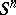of pointsof a Euclidean spacethat are situated at a constant distance(the radius of the sphere) from a point(the centre of the sphere), i.e.The sphereis a pair of points, the sphereis the circle, the sphere, when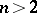, is sometimes called a hypersphere. The volume of the sphere(the length when, the surface when) is given by the formula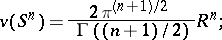in particular,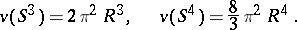Hereis the gamma-function.

The equation of a spherein the Cartesian coordinates of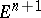takes the form(here,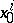,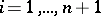, are the coordinates of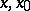respectively), i.e. the sphere is a (hyper-)quadric or a surface of the second order of special form.

The position of any point in space relative to a sphere is characterized by the power of the point (cf. Degree of a point). The totality of all spheres (in-space) relative to which a given point has a fixed power forms a web of spheres. The totality of all spheres relative to which the points of a straight line (the radical axis) have an identical power (different for different points), forms a net of spheres. The totality of all spheres relative to which the points of a plane (the radical plane) have an identical degree (different for different points), forms a pencil of spheres.

From the point of view of differential geometry, the sphereis a Riemannian space of constant curvature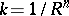. (This curvature is Gaussian whenand Riemannian when.) All geodesics of a sphere are closed and have constant length— these are known as great circles, i.e. the intersections withof two-dimensional planes inthat pass through its centre. The exterior-geometric properties ofare: all normals intersect at one point; the curvature of any normal section is one and the same and does not depend on the point at which it is examined, in particular, it has constant mean curvature, whereby the complete mean curvature of the sphere is the least among the convex surfaces of identical area; and all points of the sphere are umbilical (cf. Umbilical point).

Certain of these properties, which are taken to be fundamental, have been used as the starting point for a generalization of the concept of a sphere. For example, an affine sphere is defined by the fact that all its (affine) normals intersect at one point; a pseudo-sphere is a surface in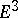of constant Gaussian curvature (although negative); one of the interpretations of a horosphere (limit sphere) is as the set of points within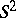defined by an equation that is also of the second degree: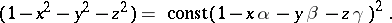The orthogonal group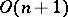of the spaceoperates doubly-transitive on(-transitivity means that for any two pairs of points with equal distances between them there is a rotation — an element of— that maps one pair onto the other); this group is the complete group of isometries of; finally, a sphere is a homogeneous space: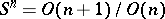.

From the point of view of (differential) topology, the sphereis a closed differentiable manifold that dividesinto two domains and that is their common boundary; the bounded domain homeomorphic tois then an (open) ball; thus, the sphere can be defined as its boundary.

The homology groups of,, are: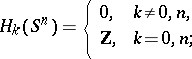in particular,does not contract into a point, i.e. the identity mapping ofonto itself is essential (cf. Essential mapping).

The homotopy groups of,, for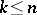are: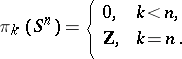In addition one has, for example,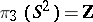, andwhen. Generally, for anyand,, the groups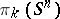have not been calculated (see Spheres, homotopy groups of the).

The concept of a sphere also has a generalization here. For example, a wild sphere is a topological sphere (see below) inthat does not bound a domain homeomorphic to; a Milnor sphere (an exotic sphere) is a manifold that is homeomorphic, but not diffeomorphic, to.

A topological space homeomorphic to a sphere is called a topological sphere. One of the basic problems here is the question of the conditions under which a space is a topological sphere.

Examples. a) No topologically invariant characterization ofwhenis known (1990). For the case where, see One-dimensional manifold. In order that a continuum be homeomorphic to the sphere, it is necessary and sufficient that it be locally connected, that it contain at least one simple closed curve and that every such curve that lies in it divides it into two domains having this curve as their common boundary (Wilder's theorem).

b) A complete simply-connected Riemannian space of dimensionwhose curvature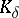for all tangent two-dimensional planesis-bounded with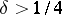, i.e.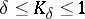, is homeomorphic to(the sphere theorem, see Riemannian geometry).

c) A simply-connected closed smooth manifold whose (integral) homology groups coincide with the homology groups ofis homeomorphic towhen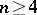(when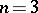, it is unknown (1990)). If, it is also diffeomorphic to(the generalized Poincaré conjecture), when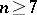, the diffeomorphism result does not hold.

A spherein a metric space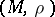is defined in exactly the same way: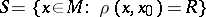. However, this set, generally speaking, may have a fairly-complicated structure (it may even be empty).

In a normed spacewith norm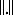, the set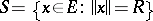is called a sphere; this is, generally speaking, essentially an arbitrary, infinite-dimensional, convex (hyper)surface, and does not always possess the properties of, for example, smoothness, roundedness and other useful properties of ordinary spheres. One of the variants used in topology — the so-called infinite-dimensional sphere — is the strict inductive limit,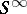, of a sequence of nested spheres: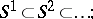another definition: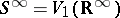, where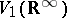is an infinite-dimensional Stiefel manifold. For any, it turns out that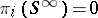.

The applications of the concept of a sphere are remarkably varied. For example, a sphere is used in constructing new spaces or supplementary structures on them. For example, the projective space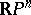can be interpreted as a spherewith diametrically-opposite points identified; a sphere with handles and holes is used in handle theory; see also Cohomotopy group; Spherical map.